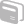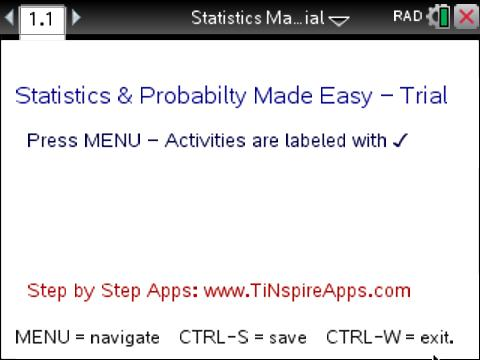# Activities

•• ##### Subject Area

• Math: Statistics: Probability and Random Variables
• Math: Statistics: Displaying and Describing Univariate Data
• Math: Statistics: Describing Bivariate Data
• Math: Statistics: Data Analysis
• Math: Statistics: Normal Distributions
• Math: Statistics: Confidence Intervals
• Math: Statistics: Inferential Statistics
• Math: Statistics: Hypothesis Tests

• ##### Author9-12
College

• ##### Activity Time

10-15 Minutes

• ##### Device
•TI-Nspire™ CX CAS/CX II CAS
• ##### Software

TI-Nspire™ CX CAS

4.2

## Statistics Made Easy - Trial Edition#### Activity Overview

In Statistics Made Easy - Trial Edition, students will use TI-Nspire technology to explore common statistics problems utilizing step-by-step processes.

#### Vocabulary

• Univariate Data
• Standard deviation
• Mean
• Median
• Mode
• Average
• Linear regression
• Normal distribution
• Bell curve
• Confidence interval

In "Statistics Made Easy- Trial Version", there are six different activities for students to explore common statistics problems, and learn how to use step-by-step processes to solve.  To access the different activities within the TNS file, from the title screen, MENU >

• Probability & Expected Value> (5) Expected Value

Compute Expected Value and Standard Deviation: Learn how to compute expected value and standard deviation by plugging in the outcomes and their probabilities in the boxes, and then viewing the steps to find its solution below.

• Statistics> (1) Find Average, Mean, and Median

Compute Mean, Mode, Median: Learn how to compute Mean, Mode, and Median by plugging in the data in the box, and viewing the steps to find its solution below.

• Regression> (2) Find regression line…

Perform Linear Regression: Learn how to perform linear regressions by plugging in the data in the boxes and viewing the steps to find its solution below.

• Distribution> (3)Normal Distribution

Perform Computations Using the Normal Distribution: Learn how to perform computations using the normal distribution by plugging in the mean and standard deviation and bounds in the boxes, and then viewing the steps to find probabilities under the bell curve below.

• Confidence Intervals> (2) CI for Mean…

Compute Confidence Interval for a Mean: Learn how to compute confidence intervals for a mean by plugging in the data in the boxes and view the steps to find its confidence interval below.

• Test of Significance> (2) Z-test for Mean…

Perform Test of Significance for a Mean: Learn how to perform test of significance by plugging in the data in the box, and viewing the steps to perform the test and draw conclusions below.

Note that all items with a check-mark next to it are functional in this trial version, so there are more activities available in the file to preview then those listed above. For more information on purchasing the full version, visit SMARTSOFT.